Study Materials

# NCERT Solutions for Class 6th Mathematics

Page 2 of 6

## Chapter 4. Basic Geometrical Ideas

### Exercise 4.2

Exercise 4.2

Q1. Classify the following curves as (i) Open or (ii) Closed.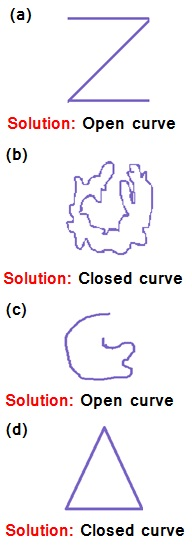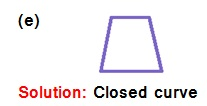Solution:

(i) Open curves : (a) and (c)

(ii) Close curves : (b) (d) and (e)

Q2. Draw rough diagrams to illustrate the following :
(a) Open curve (b) Closed curve.

Solution: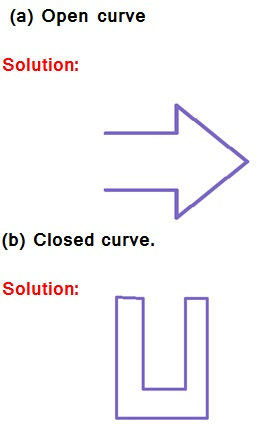Q3. Draw any polygon and shade its interior.

Solution: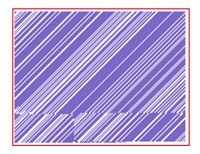Q4. Consider the given figure and answer the questions :
(a) Is it a curve? (b) Is it closed?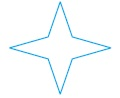Solution:

(a) Is it a curve?

Solution: yes, it is a curve

(b) Is it closed?

Solution: yes, it is a closed

Q5. Illustrate, if possible, each one of the following with a rough diagram:
(a) A closed curve that is not a polygon.
(b) An open curve made up entirely of line segments.
(c) A polygon with two sides.

Solution: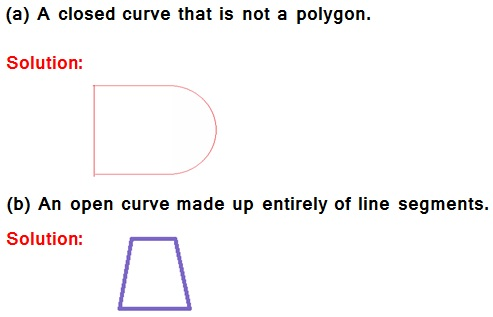(c) A polygon with two sides.

Solution:  The polygon can be made with three or more sides. There is no polygon with two sides.

Page 2 of 6

Chapter Contents: